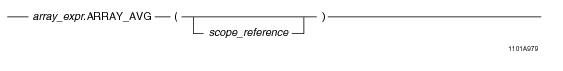# 15.00 - ARRAY_AVG - Teradata Database

## Teradata Database SQL Functions, Operators, Expressions, and Predicates

Product
Release Number
15.00
Content Type
Programming Reference
Publication ID
B035-1145-015K
Language
English (United States)

## ARRAY_AVG

### Purpose

Returns the average of all the element values in array_expr or the average of the elements within the specified scope.

### Syntax

System function syntax:Method-style syntax:where:

 Syntax element… Specifies… array_expr one of the following: A reference to an ARRAY column An ARRAY constructor expression. See “ARRAY Constructor Expression” on page 195. A UDF expression A UDM expression The element type of the array must be a numeric data type. scope_reference an optional ARRAY scope reference. See “ARRAY Scope Reference” on page 198.

### ANSI Compliance

This is a Teradata extension to the ANSI SQL:2011 standard.

### Usage Notes

ARRAY_AVG takes an array expression as an argument and returns a scalar value of type FLOAT. This value represents the result of adding the values of each element in the array argument, and dividing this result by the total number of elements. The array argument must have a numeric element type. If you specify a scope reference, the function is applied only to the elements within the limits of the given scope.

The affected elements cannot be filtered through a conditional expression. Therefore, a SELECT statement involving ARRAY_AVG must not contain an array relational expression in the WHERE clause.

If a NULL element is encountered in the array argument, it is ignored and not considered when doing the calculation. If the array argument contains any elements that is in an uninitialized state, an error is returned. Use a scope reference to avoid referencing a range of the array with uninitialized elements, or set any uninitialized elements to NULL. You can do this with the OEXTEND method. See “OEXTEND” on page 272.

If array_expr is NULL, the function returns NULL.

### Result Type

The result data type of ARRAY_AVG is FLOAT.

### Example

Consider the following 1-D ARRAY data type and table.

`CREATE TYPE item_price AS DECIMAL(7,2) ARRAY;`
` `
`CREATE TABLE inventory (itemkind INTEGER,`
`                        regular_price item_price,`
`                        sale_price item_price);`

When evaluating the following query, each element value of the regular_price array is added. The total sum of all the element values is then divided by the number of elements in the regular_price array.

`SELECT ARRAY_AVG(regular_price) FROM inventory;`

In the following query, ARRAY_AVG adds each element within the specified scope of the regular_price array. The result is a scalar value representing the total sum of adding the affected element values of regular_price divided by the total number of elements composing the specified scope.

`SELECT ARRAY_AVG(regular_price, 5, 10)FROM inventory;`

### Example

Consider the following 2-D ARRAY data type and table.

`CREATE TYPE shot_ary AS INTEGER ARRAY[1:50][1:50];`
` `
`CREATE TABLE seismic_data (`
`   id INTEGER,`
`   shot1 shot_ary,`
`   shot2 shot_ary);`

When evaluating the following query, each element value in the shot1 array is added. The total sum of all the element values is then divided by the number of elements in the shot1 array.

`SELECT ARRAY_AVG(shot1) FROM seismic_data;`

In the following query, ARRAY_AVG adds each element within the specified scope reference of the shot1 array. The result is a scalar value representing the total sum of adding the affected element values of shot1 divided by the total number of elements composing the specified scope reference.

`SELECT ARRAY_AVG(shot1, NEW arrayVec(5,5), NEW arrayVec(10,10)) `
`FROM seismic_data;`

The following is the same query using method-style syntax.

`SELECT shot1.ARRAY_AVG(NEW arrayVec(5,5), NEW arrayVec(10,10)) `
`FROM seismic_data;`